• 검색 결과가 없습니다.

# Non-equilibrium stochastic thermodynamics of Brownian particle in an active bath

N/A
N/A
Protected

Share "Non-equilibrium stochastic thermodynamics of Brownian particle in an active bath"

Copied!
80
0
0
더 보기 ( 페이지)

전체 글

The model proposed in the work reproduces the experimental results, as shown in an example of the tracer mobility in an active bath of swimming algal cells. 4 4 Poisson-correlated active power in case of  c s. a) shows active power in time, (b) represents distribution of active power. 6 5 In the limit of  c p →, the active force becomes Ornstein-Uhlenbeck noise (a) shows active force in time, (b) represents distribution of active force.

7 6 In the limit of c→0 &p →c2, the active force becomes white Gaussian noise (a) shows active force in time, (b) represents distribution of active force. 11 Mean square displacement for two extreme ratios between c and k from Eq. The MSD shows an exponentially saturating shape. 42 20 Measurement of the response function. a) The center of the trap is instantly displaced by the distance h at t=t0.

Background

## Active force modelling

The greater the concentration of the active particles, the more frequent random kicks over a period of time. The greater the mobility of the active particle, the greater the kicking force, and so is the magnitude of X. Therefore, using the Poisson property of active force and Eq. 2), the Langevin equation of the active force can be expressed as.

The active force model is made with discontinuous characteristics, but when it goes to a boundary condition, it becomes OU noise. When the stroke duration time is shorter than the Poisson interval time  c p, the PDF of the active force shows the non-Gaussian distribution as shown in Fig. When the stroke duration time is much larger than the Poisson interval time  c p →, however, the active force model becomes a continuous Gaussian correlated noise, which is the same as the OU noise model (Fig. 5).27 White Gaussian noise is also satisfied when the shock duration is very short (limit c→0) and the state of.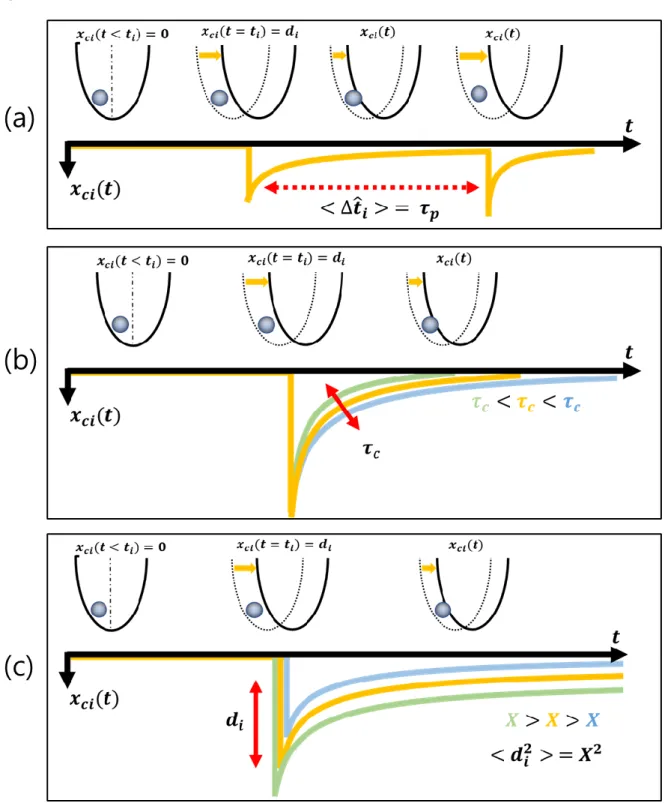Figure 3: Graphical description of three control parameters: (a) random Poisson interval time , (b) kick duration time , and (c) kick strength .

Single-laser method

Design of the single-laser method with fluorescence system

Two-Laser method

Experimental details

Computer simulation

## Poisson active force and OU noise

The PDF of the active forces has non-zero highest-order cumulants for all orders, unlike the OU noise. We can rigorously prove that our model satisfies not only Poisson correlated noise but also OU noise. Then, the forward Kolmogorov equation, which describes the probability of a given state in the stochastic process changes over time, for Eq. 3) is written up to the first order in dt as.

In the limit  =(  c p)→  the above equation becomes the Fokker Planck equation for the OU process.

Power spectral density of the particle position

Autocorrelation function

## Mean square displacement

Here Tact is Dact kB and the spread of the position at the initial time x02 can no longer use the equipartition theorem.

## Enhanced mobility with the active force

Since Tact =Dact kB, a higher active temperature means a higher active diffusion coefficient, which represents the rate of movement due to the active force alone. Since the active force is not correlated with the thermal noise32, the diffusion of the particle due to the active force is independent of the diffusion due to the thermal noise.31. In this case, the movement of the tracer particle in a short time (tc) is determined by thermal noise and active noise, and in a long time (tk) the movement is determined by the optical tracking time k .

In the short time (tk), the tracer particle is only affected by thermal noise and harmonic potential, as without an active sound environment. In the long-time tc regime, the tracer is mainly affected by the active force and the thermal force. To quantify the degree of non-Gaussianity34, we measure the non-Gaussian parameter.

This result is consistent with real bio-active experiments in which the PDF shows a non-Gaussian distribution when the concentration of active particles in the surrounding medium is low, corresponding to  c p 1.7 The detailed comparison is in Section 4.3. One is the xth  −x xact motion caused by thermal noise and the other is the xact motion caused by the active force. It is caused by the equilibrium process of the particle position, located within the harmonic potential that erases the non-Gaussian effect of the active noise.

Interestingly, under these conditions, although ( )P x exhibits a Gaussian distribution, the van Hove self-correlation function Gs( x, t), which is the PDF of the particle displacement x during t, exhibits a non- Gaussian distribution on a shorter time regime in Fig.13. Currently, the non-Gaussian tail is explained by theory taking into account the hydrodynamic interactions between the tracer particle and the active particles. This conditional PDF of x for xact( )t is Gaussian, while the PDF averaged over xact( )t becomes non-Gaussian in general.

Complete statistics require information on all higher-order moments due to the non-Gaussian property of xact, which is practically impossible.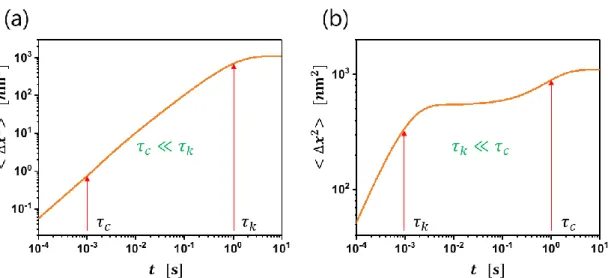Figure 11: Mean square displacement for two extreme ratio of and from Eq. (44). (a) In the case of , the MSD shows an exponentially saturating form

## Real bio-active vs. active force model

In a short time case, there is not enough time for the tracer particle to feel the active force. According to the central limit theorem, the tracking behavior shows Brownian motion in a long time regime. However, when the time scale is close to the kick duration time tc, the MSD shows superdiffusion as x t2( ) t with 1, because the active force boosts the mobility of the tracer in this time scale.

Pigeon (colored hollow circles) when the random impact force is fRMS =0.4 pN and the average impact interval time corresponds to the ratio p −3 2. Here, N is the number of active cells, Vc is the average cell volume, and rc is the average cell radius . According to our model, we assume that the concentration of active particles is inversely proportional to the average time of the impact interval p.

Since the average time interval between kicks p is related to the concentration of the cell, the p is expressed as p −1 −3 2. We find that when there are many collision events between the active cells and the tracer particles as due to the high cell concentration,. If we assume that the diffusion is dominated by the active particle, Deff =Dth+Dact Dact.

This explains that the simulation using our model agrees well with the experimental data as shown in Fig.18 (a). To realize that the random impact force fRMS=400 Nn, we assumed that the instantaneous velocity of the tracer particle is the average velocity of the cell when the cell interacts with the tracer particle. In this case, the average drag force felt by the tracer particle is the satisfying Stokes force.

The above comparison between the real bioactive substance system and the active force model suggests that although our active force model does not include the mechanics of the active bath in detail.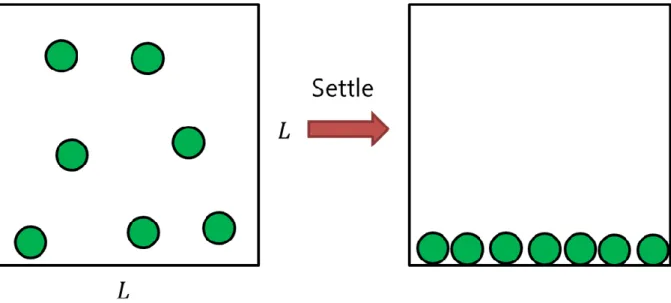Figure 17: N number of swimming active cells in the 3D box of length . Here, the green circles represent each cell

## Heat dissipation in an active bath

• Violation of Fluctuation-dissipation theorem in active bath
• Power spectral density of position
• Velocity autocorrelation function C ( )
• Response function of the system R ( )
• Quantifying the heat dissipation rate
• Quantifying the work production rate
• Maximum heat dissipation rate frequency

Harada and Sasa45 showed how to quantify the rate of heat dissipation under non-equilibrium conditions, known as the Fluctuation-Response Relationship (FRR) which can be derived from Eq. Here, vs is the steady-state velocity of the system, C( ) is the velocity auto-correlation function, and R(. Note that the response function is independent of the active force as well as the thermal force.

In frequency space  the system response function R( ). Figure 20: Measurement of the response function. a) The center of the trap is immediately shifted with increasing distance. The tracer particle trajectories are averaged over 500 different measurements under the same conditions. However, when the system is not in equilibrium due to the presence of the active force, FDT is violated.

The response function does not change as long as the active force does not affect the internal properties of the system.47 Here, D( ) represents the frequency-dependent rate  of FDT violation, which is random to the rate of heat dissipation. Here, the heat dissipation rate is proportional to the active temperature Takti and inversely proportional to the stroke duration time c. V t =k x− t k and the work speed W is equal to − −(x act( )t k)act( ).t The second is from the point of view that the particle also feels the fixed harmonic potential kx2 2 and the active potential x act( )t.

Here, V t( )=kx2 2−xact( )t and the work rate is expressed as W = −xact( ).t The third is to consider the random active force as an external force depending on time and the system feels only temporal independent harmonic potential. Therefore, we can find the frequency of maximum heat dissipation rate d using D and Eq. Therefore, it is found that the maximum rate of heat dissipation is at a certain frequency i.e. 1.

Therefore, it is not surprising that the time scale characterizing the maximum heat dissipation is the geometric mean of k and c.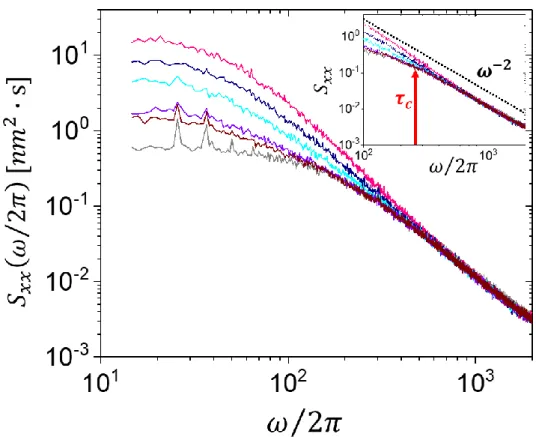Figure 19 shows the power spectral density of position in the experiment with various conditions

Simulation code (Matlab)

The time-correlation function of active forces

## Work rate

Work rate (1)

Work rate (2)

### Work rate (3)

In summary, we studied the non-equilibrium stochastic thermodynamic picture of the active matter system. Our model is defined by three parameters: the average time interval between shocks p which is related to the active particle concentration, the shock duration c which is related to the characteristic time of the active particle, and the shock strength X which is related to the random shock intensity. In the experiment, a tracer particle is surrounded by the environment created by the thermal and active baths.

Furthermore, the particle position probability density distribution is no longer Gaussian, except when the average time interval between kicks is much shorter than the kick duration. To test the model in real bioactive systems, we performed a computer simulation with our model and compared it with a previously published experiment. We found that the rate of heat dissipation in the experiment is proportional to the active temperature (activity) of the tracer particles and inversely proportional to the correlation time of the active particle in steady state.

The experimentally measured heat dissipation rate agrees with the steady-state operating rate derived analytically. It can be applied to Brownian objects in different types of active baths in both computer simulations and experimental measurements. Different models have been proposed in the literature to describe such behavior - "strange kinetics of diffusive diffusivity",49 random walks in continuous time,50 phenomenology,5, 51 and many more.

Although these features can in principle be addressed using the active model introduced here, they are beyond the scope of the current study. Maggi, C.; Paolozzi, M.; Angelani, L.; Di Leonardo, R., Memoryless response and violation of the fluctuation-dissipation theorem in colloids suspended in an active bath. Bellon, L.; Ciliberto, S.; Laroche, C., Violation of the fluctuation-dissipation relation during the formation of a colloidal glass.

Dieterich, E.; Camunas-Soler, J.; Ribezzi-Crivellari, M.; Seifert, U.; Ritort, F., Single molecule measurement of the effective temperature in non-equilibrium steady states.

수치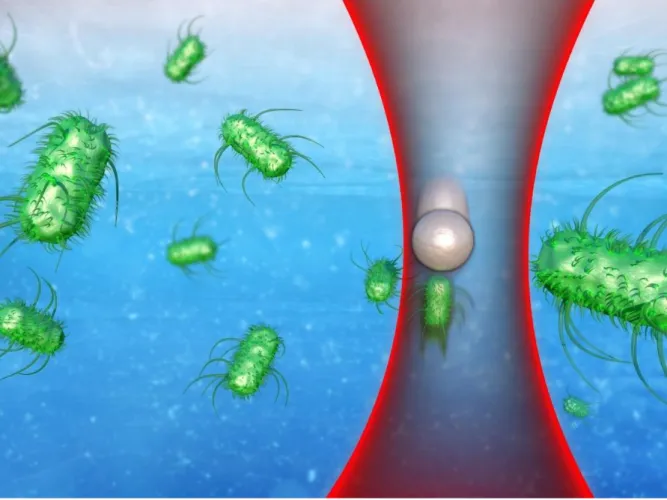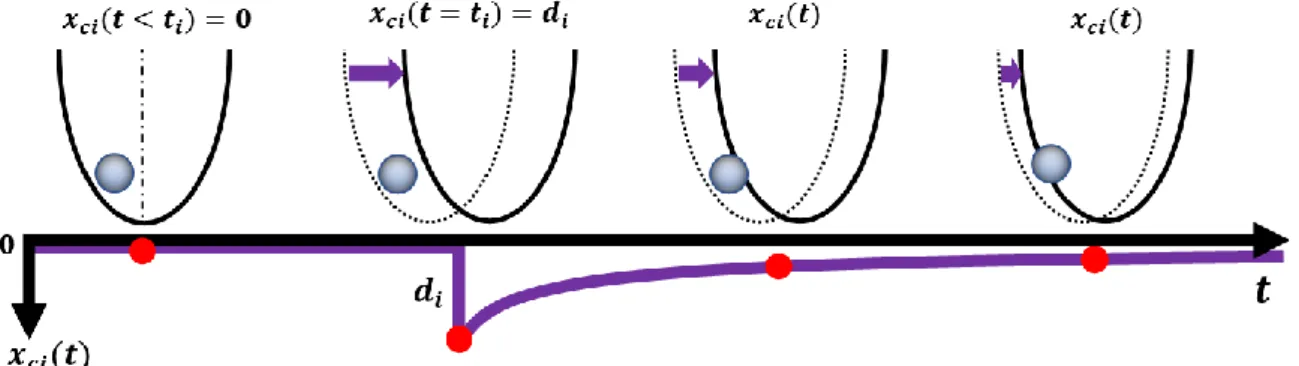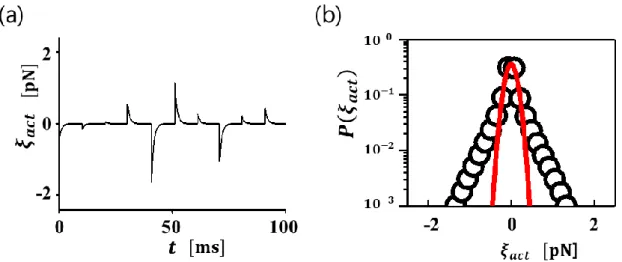+7

참조

관련 문서

ues.30 The designations, drop-MO(nc/nv) and drop-MO(nc), are used to represent that the nc innermost occupied and the nv outermost virtual molecular orbitals are dropped in the

Changes in Snow Depth Variability over Northeast Asia: Observed and Projections through Coupled Climate Models.. Ramesh Kripalani 1 , Jai‐Ho

The kick-o ﬀ method is based on an OLS estimator that is not robust to outliers in the data. To overcome this problem, we have modified the kick-o ﬀ approach based on the

Most absorption analysis has been based on odor intensity. An essential oil sufficiently neutralized with chemical functio- nal group of terpenes relative to ammonia as shown

n The magnitude of the buoyant force acting on a floating body in the fluid is equal to the weight of the fluid which is displaced by

This report assesses selected structural reforms in product markets in Italy, Spain, Portugal and Greece, although the econometric estimates presented can easily be applied to

Because these collisions are random, in the long run the total force acting on the particle is zero, but at any one time there will be more collisions on one side of the particle

People Services Branch Aboriginal and Torres Strait Islander Reference Group Reconciliation Champions Youmpla Network SES.. We encourage and support

the United States should look favorably on all measures that would promote growth and employ- ment by genuinely increasing economic freedom, but it should not accept any

언어 선택하기

웹사이트는 선택한 언어로 번역됩니다.

기타 언어